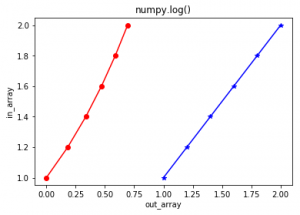GeeksforGeeks App
Open AppBrowser
Continue

The numpy.log() is a mathematical function that helps user to calculate Natural logarithm of x where x belongs to all the input array elements. Natural logarithm log is the inverse of the exp(), so that log(exp(x)) = x. The natural logarithm is log in base e.

Syntax :numpy.log(x[, out] = ufunc ‘log1p’) Parameters : array : [array_like] Input array or object. out : [ndarray, optional] Output array with same dimensions as Input array, placed with result. Return : An array with Natural logarithmic value of x; where x belongs to all elements of input array.

Code #1 : Working

## Python3

 `# Python program explaining``# log() function``import` `numpy as np` `in_array ``=` `[``1``, ``3``, ``5``, ``2``*``*``8``]``print` `("``Input` `array : ", in_array)` `out_array ``=` `np.log(in_array)``print` `("Output array : ", out_array)`  `print``("\nnp.log(``4``*``*``4``) : ", np.log(``4``*``*``4``))``print``("np.log(``2``*``*``8``) : ", np.log(``2``*``*``8``))`

Output :

```Input array :  [1, 3, 5, 256]
Output array :  [ 0.          1.09861229  1.60943791  5.54517744]

np.log(4**4) :  5.54517744448
np.log(2**8) :  5.54517744448```

Code #2 : Graphical representation

## Python3

 `# Python program showing``# Graphical representation ``# of log() function``import` `numpy as np``import` `matplotlib.pyplot as plt` `in_array ``=` `[``1``, ``1.2``, ``1.4``, ``1.6``, ``1.8``, ``2``]``out_array ``=` `np.log(in_array)` `print` `("out_array : ", out_array)` `plt.plot(in_array, in_array,``         ``color ``=` `'blue'``, marker ``=` `"``*``")` `# red for numpy.log()``plt.plot(out_array, in_array,``         ``color ``=` `'red'``, marker ``=` `"o")``         ` `plt.title("numpy.log()")``plt.xlabel("out_array")``plt.ylabel("in_array")``plt.show()`

Output :

`out_array :  [ 0.          0.18232156  0.33647224  0.47000363  0.58778666  0.69314718]`References : https://docs.scipy.org/doc/numpy-dev/reference/generated/numpy.log.html#numpy.log .

numpy.log() is a function in the NumPy library of Python that is used to calculate the natural logarithm of a given input. The natural logarithm is a mathematical function that is the inverse of the exponential function. The function takes an array or a scalar as input and returns an array or a scalar with the natural logarithm of each element.

### Advantages of using numpy.log() function in Python:

1. Speed: numpy.log() function is highly optimized for fast computation, which makes it suitable for handling large datasets and complex calculations in scientific computing and data analysis.
2. Mathematical accuracy: numpy.log() function provides high mathematical accuracy for calculating natural logarithms, which makes it useful in numerical simulations and scientific experiments.
3. Versatility: numpy.log() function can be used with a wide range of input types, including scalars, arrays, and matrices.
4. Integration with other NumPy functions: numpy.log() function can be easily integrated with other NumPy functions and libraries, allowing for more complex calculations and data analysis.

### Disadvantages of using numpy.log() function in Python:

1. Limited domain: numpy.log() function is only defined for positive real numbers, and will raise a ValueError if given a non-positive number.
2. Limited functionality: While numpy.log() function is useful for calculating natural logarithms, it has limited functionality compared to other more specialized libraries and functions for mathematical operations and data analysis.
3. Requires NumPy library: To use numpy.log() function, you need to have the NumPy library installed and imported in your Python environment, which can add some overhead to your code and may not be suitable for certain applications.

### Here are some important points to keep in mind while using numpy.log() function in Python:

1. The numpy.log() function calculates the natural logarithm of a given input.
2. The natural logarithm is a mathematical function that is the inverse of the exponential function.
3. The function takes an array or a scalar as input and returns an array or a scalar with the natural logarithm of each element.
4. The numpy.log() function is highly optimized for fast computation, making it suitable for handling large datasets and complex calculations in scientific computing and data analysis.
5. The numpy.log() function can be used with a wide range of input types, including scalars, arrays, and matrices.
6. The numpy.log() function is only defined for positive real numbers, and will raise a ValueError if given a non-positive number.
7. The numpy.log() function provides high mathematical accuracy for calculating natural logarithms, making it useful in numerical simulations and scientific experiments.
8. To use the numpy.log() function, you need to have the NumPy library installed and imported in your Python environment.

### If you’re looking for a reference book

on NumPy, one popular option is “Python for Data Analysis” by Wes McKinney. This book covers NumPy in depth, along with other important Python libraries for data analysis such as pandas and matplotlib. It also includes practical examples and exercises to help you apply what you learn.

My Personal Notes arrow_drop_up